## Wednesday, October 28, 2009

### Excel: too many adjustable cells

> My Excel Solver model gives this error message:

Yes, this means Solver has hit the built-in limit of 200 variables. See: http://office.microsoft.com/en-us/excel/HP100738491033.aspx. You can upgrade to a solver from Frontline, or if you want a more structured Excel based approach consider something like Microsoft Solver Foundation. Also Cplex 12 has an Excel interface much like Solver. All the other modeling languages also have Excel interoperability.

Apparently, there are quite a few models with fewer than 200 variables, see http://www.utexas.edu/courses/lasdon/design3.htm.

Note that for some models the 200 variable limit is less restrictive than it seems as intermediate variables do not have to be included in the adjustable cells.

For larger models I am a big proponent of moving to a modeling language. I have converted a number of models written in Excel to GAMS and AMPL and actually in virtually all of them I had problems in reproducing the results: all these Excel spreadsheets contained bugs. It is just too difficult to do error-free comprehensive modeling using the Excel formula paradigm. I’d suggest to use Excel where it is really good: as data editor and for reporting. For the stuff in between: the actual modeling, nothing beats a specialized modeling language.

### NLP infeasible

A client of mine sent me a large NLP (36k variables and equations) that was infeasible. When a general local NLP solver returns INFEASIBLE, this may mean two things:

1. The model is infeasible, i.e. no feasible solution exists
2. or the model contains some feasible solutions but the solver cannot find any

As is the case for many NLP models this model had many linear equations. So I first solved for the linear equations only using an LP solver. This was still infeasible. Now we know we have case 1. After fixing this problem (some bounds were too tight) I kept the LP in, as with little effort I could construct a feasible solution for the whole problem using the LP solution. The NLP solver I used was CONOPT which is a feasible path algorithm, so it will stay feasible when the initial point is feasible. This trick may speed up the solution procedure but also provides for better results in case the solver stops prematurely: it will give us at least a solution that is feasible.

## Tuesday, October 27, 2009

### Tables in Excel 2007

I started to convert some spreadsheets to Excel 2007. This will allow me to use Tables. See e.g. http://www.jkp-ads.com/Articles/Excel2007Tables.asp, http://blogs.msdn.com/excel/archive/2005/10/28/486604.aspx. Need to remember this word: “structured referencing”.

## Saturday, October 24, 2009

### Is there a way to convert this linear functional optimization problem into linear programming?

max a'*x / b'*x

w.r.t x,

s.t. x>=0, 1'*x=1

Here a and b are given vectors, " ' " denotes the vector
transposition, x is our search variable which is a vector, 1 is also a
vector above. It means the element of x should sum up to 1.

Any thoughts?

Thanks a lot!

Sometimes. In slightly different notation, your model looks like: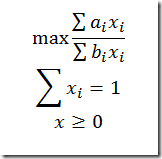We need to think about the denominator becoming zero. If we allow any b(i) this can easily happen. One possible way to make the problem well defined is to consider b(i)>0. (Alternatively all b(i)<0 will do). So let’s assume all b(i)>0.

First of all, this problem is not that difficult to solve for a general NLP solver. Good old MINOS is very quick on this even for large instances (e.g. 10k variables). The Hessian becomes big then, so solvers requiring second derivatives are in a disadvantage here. Note: there is a reformulation possible that reduces the size of the Hessian: write the objective as constraint of the form varobj*sum(i, b(i)*x(i))=sum(i,a(i)*x(i)).

However, there is a reformulation possible into an LP.  When we apply the transformation: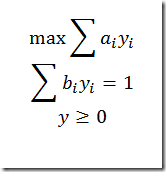After solving we can recover the x variables through: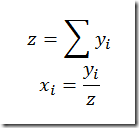For more info about this transformation see section 4.3.2 in http://www.stanford.edu/~boyd/cvxbook/bv_cvxbook.pdf or section 2.10.2 in http://www.dashoptimization.com/home/downloads/book/booka4.pdf. I simplified things a little bit by using that x(i) sums to 1. The disadvantage of this transformation for more complex models is that we really need to change the complete model.

Alternatively we may exploit the structure of the model and just use the simple algorithm: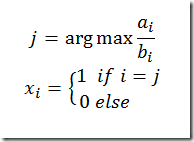So we don’t even need linear programming here, just find the maximum of n numbers. The above is illustrated in the following GAMS model:

*------------------------------------------------------
* random data, b(i)>0
*------------------------------------------------------

set i /i1*i10000/;
parameter a(i), b(i);
a(i) = uniform(-10,10);
b(i) = uniform(1,10);

*------------------------------------------------------
* nonlinear model
*------------------------------------------------------

positive variable x(i) 'nlp solution';
variable obj1;
equations e_obj1, sum1;

e_obj1.. obj1 =e=
sum(i, a(i)*x(i))/sum(i, b(i)*x(i));
sum1..
sum(i, x(i)) =e= 1;

* initial point
x.l(i) = 1/
card(i);

model m1 /e_obj1,sum1/;
solve m1 maximizing obj1 using nlp;

*------------------------------------------------------
* transformed linear model
*------------------------------------------------------

positive variable y(i);
variable obj2;
equations e_obj2, sum2;

e_obj2.. obj2 =e=
sum(i, a(i)*y(i));
sum2..
sum(i, b(i)*y(i)) =e= 1;

model m2 /e_obj2,sum2/;
solve m2 maximizing obj2 using lp;

*------------------------------------------------------
* recover x
*------------------------------------------------------

parameter pz,px(i) 'recovered solution';
pz =
sum(i,y.l(i));
px(i) = y.l(i)/pz;

display x.l,px;

*------------------------------------------------------
* alternative algorithm
*------------------------------------------------------

parameter c(i); c(i) = a(i)/b(i);
scalar max; max = smax(i, c(i));
display max;
set jmax(i); jmax(i)\$(c(i)=max) = yes;
abort\$(card(jmax)<>1) "want a single max a(i)/b(i)";
parameter px2(i) 'alternative solution';
px2(jmax) = 1;
display px2;

## Wednesday, October 21, 2009

### MIQP

I am following your blog with great interest and I would be very happy to have your insight on an optimisation problem I am currently struggling with.
I am wondering if there is an easy way to solve a model with linear constraints on a vector of both continuous and binary variables and a quadratic objective function depending only on the subset of continous variables (e.g. least squares distance to an objective vector) without having to resort to a non-linear solver.
I reckon this is some kind of 'MIP + Constrained' Linear Least Squares. I would be disappointed if there was no better way to solve this than treating it as 'any' non-linear problem, but as I found nothing relevant at the moment, I'd be glad to hear what you think about it.

Some solvers (such as Cplex) offer a MIQP (Mixed Integer Quadratic Programming) functionality. That may be something to try out. Otherwise, if the objective tries to penalize some large deviations, it may be possible to achieve a similar result by minimizing the maximum deviation. This can be formulated in a linear fashion.

Yet another linear approximation can be formed by minimizing the sum of absolute deviations. For an example see http://yetanothermathprogrammingconsultant.blogspot.com/2009/07/ms-solver-foundation-excel-interface_07.html. These linear formulations essentially choose a different norm ||x||.

### Informs powerpoint

Powerpoint from INFORMS presentation Data and Software Interoperability with GAMS at San Diego INFORMS meeting.

Basic ideas:

1. Specialized Modeling Languages such as AMPL and GAMS are in many cases more efficient than using traditional programming languages, even when fortified with a modeling API (Ilog Concert or MS SFS). It is unfortunate that those environments are getting as much attention as they do.
2. GAMS/GDX is a tool that really works. Some large scale examples are shown.
3. Problems in the interface between systems (e.g between modeling language and database) are to be expected. These are the seams of an application, and here is where problems are more likely to occur. This is unfortunate because fixing these problems is more difficult: you need knowledge about both systems e.g. SQL and GAMS to debug. This is an extension of Murphy’s law: bugs appear where they are most difficult to debug and fix.
4. SQL2GMS/MDB2GMS is less elegant than direct access through specialized syntax as in AMPL. However the big advantage is that we can repair problems on-the-fly using SQL. SQL is typically good in fixing problems where GAMS is less well-suited (e.g. string processing). In practice almost all data import requires some fixing.
5. GAMS is one of the modeling languages that is very well equipped to do data manipulation. For some policy evaluation models this is very important. Some numerical evidence is given about such models: large models with > 95% of the code dedicated to data manipulation. GAMS excels when dealing with sparse data.
6. New facilities in GAMS allow to call external programs and exchange data through GDX. This works but can be cumbersome. A proposal is presented to allow for direct function calls to external functions.

## Tuesday, October 20, 2009

### NLP: bilinear terms

Local NLP solvers have often problems with terms z=x × y, x,y ≥ 0. There are some quick and dirty tricks that can help.

• Provide a starting point <> 0. When starting from 0 a solver may not even move because of the zero gradients.
• Replace the lower bound of 0 by a small nonzero value. That will prohibit these dangerous zero values. Alternatively write
• z=(x+ε) × (y+ε), x,y ≥ 0 where ε>0 is a small constant.

There are some more sophisticated reformulations (see e.g. Liberti, Pantelides) but as first line of attack, the above may be helpful.

## Saturday, October 17, 2009

### What is a .GCH file?

In the picture below from your blog I see a .GCH file. What is that?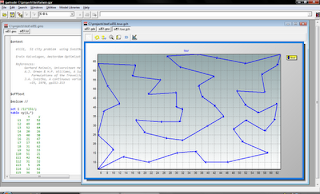That is a file with instructions for the graphics module in the GAMS IDE. It is a text file so you can look at it. The IDE can view it as text or show the results. For more information have a look at the help in IDE: HelpGAMSIDE Help TopicsGuided TourCreating Charts.

## Thursday, October 15, 2009

### Data Manipulation vs. Model Equations

In some Policy Evaluation models the ratio between lines of code (loc) doing data manipulation vs. loc implementing model equations can be surprisingly large. For these models it helps that GAMS has excellent facilities for doing data manipulations (the most important is probably the DISPLAY statement). Here is some numerical evidence:

These are all GAMS models. The Impact2000 model has actually no SOLVE statement, i.e. it is only data manipulation.

## Thursday, October 8, 2009

### Microsoft Solver Foundation v2

MSF v2 is out. See http://code.msdn.microsoft.com/solverfoundation/. Some of the new features:

• Improved Excel plugin
• Stochastic Programming support
• SOCP support
• More CSP features
• Gurobi v2 included

Some of my bigger models solve significantly faster!

## Saturday, October 3, 2009

### Gurobi v2

Gurobi v2 is out. The MIP performance has much improved and the web site is worth checking out. There are a few interesting nuggets to be found there:

• pay-per-view type of licensing through the Amazon cloud.